### 9.4.1.2 Randomized Nash equilibria

What happens if a game has no Nash equilibrium over the space of deterministic strategies? Once again the problem can be alleviated by expanding the strategy space to include randomized strategies. In Section 9.3.3 it was explained that every zero-sum game under Formulation 9.7 has a randomized saddle point on the space of randomized strategies. It was shown by Nash that every nonzero-sum game under Formulation 9.8 has a randomized Nash equilibrium . This is a nice result; however, there are a couple of concerns. There may still exist other admissible equilibria, which means that there is no reliable way to avoid regret unless the players collaborate. The other concern is that randomized Nash equilibria unfortunately cannot be computed using the linear programming approach of Section 9.3.3. The required optimization is instead a form of nonlinear programming [94,664,731], which does not necessarily admit a nice, combinatorial solution.

Recall the definition of randomized strategies from Section 9.3.3. For a pair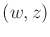of randomized strategies, the expected costs,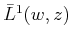and, can be computed using (9.57). A pair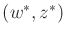of strategies is said to be a randomized Nash equilibrium if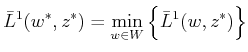(9.71)

and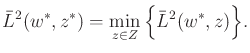(9.72)

In game-theory literature, this is usually referred to as a mixed Nash equilibrium. Note that (9.71) and (9.72) are just generalizations of (9.66) and (9.67) from the space of deterministic strategies to the space of randomized strategies.

Using the cost matrices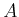and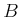, the Nash equilibrium conditions can be written as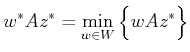(9.73)

and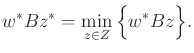(9.74)

Unfortunately, the computation of randomized Nash equilibria is considerably more challenging than computing saddle points. The main difficulty is that Nash equilibria are not necessarily security strategies. By using security strategies, it is possible to decouple the decisions of the players into separate linear programming problems, as was seen in Example 9.16. For the randomized Nash equilibrium, the optimization between the players remains coupled. The resulting optimization is often referred to as the linear complementarity problem. This can be formulated as a nonlinear programming problem [664,731], which means that it is a nonlinear optimization that involves both equality and inequality constraints on the domain (in this particular case, a bilinear programming problem is obtained ).

Example 9..20 (Finding a Randomized Nash Equilibrium)   To get an idea of the kind of optimization that is required, recall Example 9.18. A randomized Nash equilibrium that is distinct from the two deterministic equilibria can be found. Using the cost matrices from Example 9.18, the expected cost for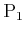given randomized strategies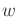and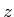is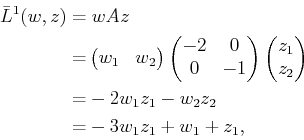(9.75)

in which the final step uses the fact that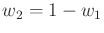and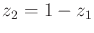. Similarly, the expected cost for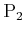is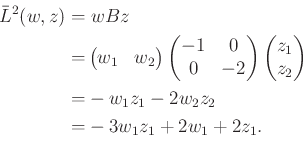(9.76)

Ifis fixed, then the final equation in (9.75) is linear in; likewise, ifis fixed, then (9.76) is linear in. In the case of computing saddle points for zero-sum games, we were allowed to make this assumption; however, it is not possible here. We must chooseto simultaneously optimize (9.75) while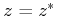and (9.76) while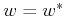.

It turns out that this problem is simple enough to solve with calculus. Using the classical optimization method of taking derivatives, a candidate solution can be found by computing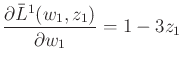(9.77)

and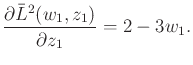(9.78)

Extrema occur when both of these simultaneously become 0. Solving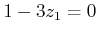and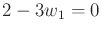yields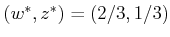, which is a randomized Nash equilibrium. The deterministic Nash equilibria are not detected by this method because they occur on the boundary of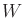and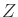, where the derivative is not defined.The computation method in Example 9.20 did not appear too difficult because there were only two actions per player, and half of the matrix costs were 0. In general, two complicated equations must be solved simultaneously. The expressions, however, are always second-degree polynomials. Furthermore, they each become linear with respect to the other variables iforis held fixed.

Subsections
Steven M LaValle 2020-08-14